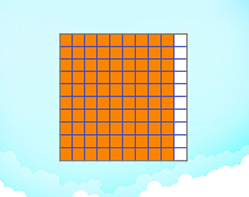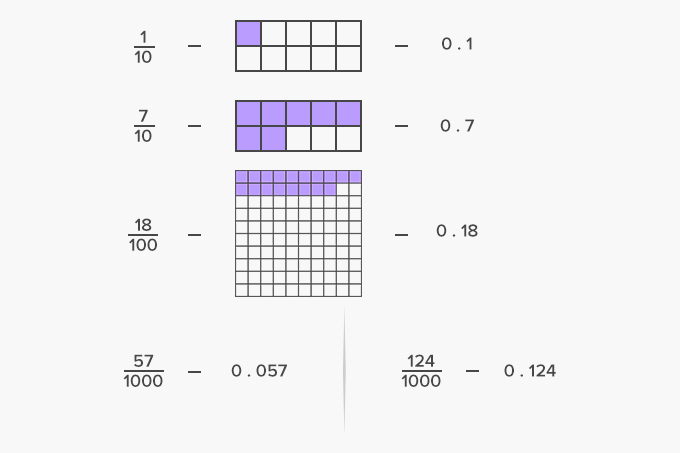# Decimal Fraction - Definition with Examples

The Complete K-5 Math Learning Program Built for Your Child

• 40 Million Kids

Loved by kids and parent worldwide

• 50,000 Schools

Trusted by teachers across schools

• Comprehensive Curriculum

Aligned to Common Core

## Decimal Fraction GamesDecimals and Fractions - Tenths

Write fractions with denominator 10 (tenths) as decimal numbers and write decimals as tenths. For example, 8 tenths = 0.8 = 8/10

Covers Common Core Curriculum 4.NF.6

## What is a decimal fraction?

In algebra, a decimal fraction is a fraction whose denominator is 10 or a multiple of 10 like 100, 1,000, 10,000, etc.Fun Facts Decimal fractions were first developed and used by the Chinese in the end of 4th century BC, and then spread to the Middle East and from there to Europe.

##Let's do it!

Instead of teaching decimal fractions and then handing out practice worksheets to your children, ask them to find the make decimals fractions of the decimals or fractions you say.

##Related math vocabulary

Won Numerous Awards & Honors